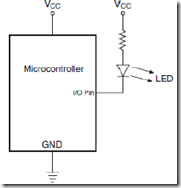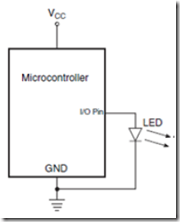" /> Microcontroller Interface with LED and Rele - TN Mikro
Home > English > Microcontroller Interface with LED and Rele

# Microcontroller Interface with LED and Rele

Connect the parallel port with LED
General configuration for connecting an LED to a microcontroller is as shown in Figure 1:21 (a) LED lights up when the pin microcontroller given sinya LOW and will die when the pin microcontroller is HIGH. Prisoners are mounted on angkaian used to limit the current flowing through the LED.
Resistance value is determined by the equation
R = (VCC – VLED) / I

Where VLED is the voltage across the LED and the first current flowing through the LED.
In general VLED and I is 1.5 V and 20 mA. If the current source microcontroller enough ability to directly control the LED, the LED connected to the microcontroller as shown in Figure 1:21 (b). In this case the LED will light when the pin on the microcontroller is HIGH.Figure 21 (a) Active LowFigure 22 (b) Active High

Interfaces with Rele
Figure 1:22 shows the connection diagrams for relay interface with a microcontroller. NPN transistor is used to provide the desired current to the relay coil because the microcontroller can not be directly connected to the microcontroller pins. When the pin microcontroller in the set HIGH transistor will be ON, then a current flows through the coil and contact will be closed. When the microcontroller pin is LOW, transistor OFF and the inductor current now flowing through the diode will drop to zero and the contact will open.Figure 23 Interface relay with microcontroller